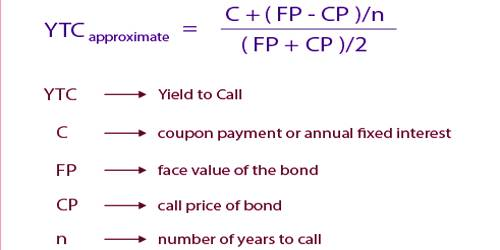# Yield to Call

Yield to Call: The decision to call (or to refund) the issue is the effect of a potential call on a bond’s expected rate of return. If the going rate of interest is well below a callable bond’s coupon rate, then the bond is likely to be called. It is a measure of the yield of a bond if you were to hold it until the call date. It implies that the bond will be redeemed at the call date before the full maturity. The calculation of yield to call is based on the coupon rate, the length of time to the call date and the market price.

The components of the formula are as follows:

• P = the current market price
• C = the annual coupon payment
• CP = the call price
• t = the number of years remaining until the call date
• YTC = the yield to call

The complete formula to calculate yield to call is:

P = (C/2) x {(1 – (1 + YTC / 2) ^ -2t) / (YTC / 2)} + (CP / (1 + YTC / 2) ^ 2t)

In this case, investors should estimate the expected of return on the bond as the yield to call (YTC) rather than yield to maturity. For most bond investors, it is significant to also estimate the yield to call, or the total return that would be received if the bond purchased was held until its call date instead of full maturity. Example: You can only estimate yield to call (YTC), as you really have no idea when or if the issuer will issue a call. For example, a 10-year bond may be callable after 4 years, so your calculation would include the 4-year date. So, it is the discount rate that makes the present value of cash inflows to call equal to the bond’s current market price.